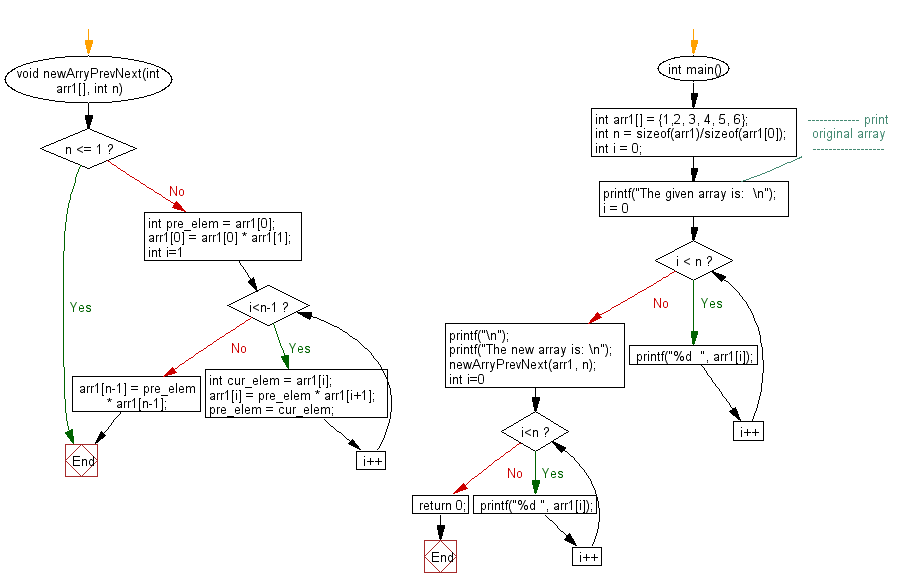﻿ C exercises: Update every array element with multiplication of previous and next numbers in array - w3resource# C Exercises: Update every array element with multiplication of previous and next numbers in array

## C Array: Exercise-103 with Solution

Write a program in C to update every array element with multiplication of previous and next numbers in array.

Sample Solution:

C Code:

``````#include<stdio.h>
void newArryPrevNext(int arr1[], int n)
{
if (n <= 1)
return;
int pre_elem = arr1;
arr1 = arr1 * arr1;
for (int i=1; i<n-1; i++)
{
int cur_elem = arr1[i];
arr1[i] = pre_elem * arr1[i+1];
pre_elem = cur_elem;
}
arr1[n-1] = pre_elem * arr1[n-1];
}
int main()
{
int arr1[] = {1,2, 3, 4, 5, 6};
int n = sizeof(arr1)/sizeof(arr1);
int i = 0;
//------------- print original array ------------------
printf("The given array is:  \n");
for(i = 0; i < n; i++)
{
printf("%d  ", arr1[i]);
}
printf("\n");
//-----------------------------------------------------------
printf("The new array is: \n");
newArryPrevNext(arr1, n);
for (int i=0; i<n; i++)
printf("%d ", arr1[i]);
return 0;
}
```
```

Sample Output:

```The given array is:
1  2  3  4  5  6
The new array is:
2 3 8 15 24 30
```

Pictorial Presentation:Flowchart:C Programming Code Editor:

Improve this sample solution and post your code through Disqus.

﻿

## C Programming: Tips of the Day

Where is the C auto keyword used?

auto is a modifier like static. It defines the storage class of a variable. However, since the default for local variables is auto, you don't normally need to manually specify it.

Ref : https://bit.ly/3yzwC9r